# Test Prep Plan - Take a practice test

Take this practice test to check your existing knowledge of the course material. We'll review your answers and create a Test Prep Plan for you based on your results.
How Test Prep Plans work
1
2Based on your results, we'll create a customized Test Prep Plan just for you!
3Study smarter
Study more effectively: skip concepts you already know and focus on what you still need to learn.

# Overview of Trigonometry: Help and Review Chapter Exam

Exam Instructions:

Choose your answers to the questions and click 'Next' to see the next set of questions. You can skip questions if you would like and come back to them later with the yellow "Go To First Skipped Question" button. When you have completed the practice exam, a green submit button will appear. Click it to see your results. Good luck!

### Page 1

#### Question 1 1. What is x?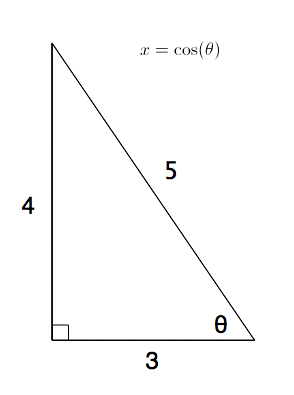#### Question 2 2. In the pictured triangle, ∠A is 62 degrees and ∠C is 86 degrees. If side a is 112 units long, approximately how long is side c?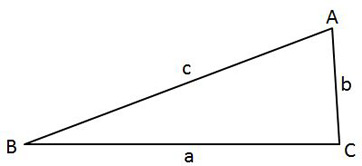#### Question 4 4. In the pictured triangle, side a is 17 and side c is 20. If ∠B is 19 degrees, then approximately how long is side b? Round the value of the cosine to the nearest whole number.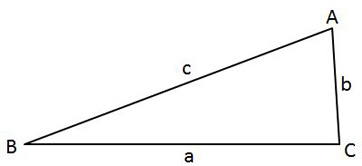#### Question 5 5. Simplify the following expression. Report the answer in degrees.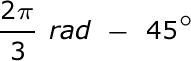### Page 2

#### Question 8 8. The lines a and b are parallel lines of length 5 and 2. The lines d and e are parallel to one another, and both are perpendicular to a and b. What is the length of c?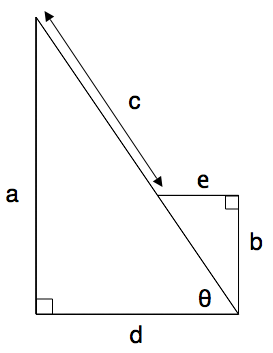#### Question 9 9. Solve the following trigonometric equation for x.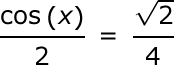#### Question 10 10. What's the value of 2sin(θ)cos(θ) given that the equation below is true?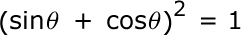### Page 3

#### Question 11 11. In the pictured triangle, ∠A is 137 degrees and ∠B is 28 degrees. If side b is 71 units long, approximately how long is side a?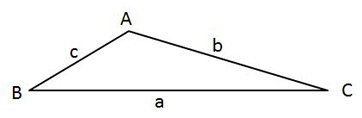#### Question 12 12. Convert the following measurement in radian to degrees.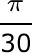#### Question 14 14. What is x?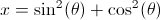#### Question 15 15. In the pictured triangle, ∠A is 98 degrees and ∠B is 12 degrees. If side a is 84 units long, approximately how long is side b?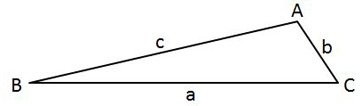### Page 4

#### Question 17 17. Solve for the value of x in the trigonometric equation below. (Use the domain -π/2 to π/2)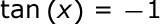#### Question 18 18. What is x?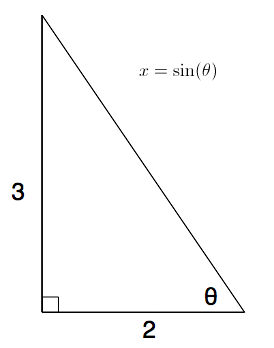#### Question 19 19. In the pictured triangle, side b is 12 and side c is 16. If ∠A is 84 degrees, then approximately how long is side a? Round the value of the cosine to the nearest whole number.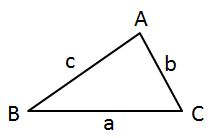#### Question 20 20. Simplify the following expression.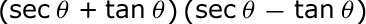### Page 5

#### Question 21 21. In the pictured triangle, side a is 9 and side b is 6. If ∠C is 26 degrees, then approximately how long is side c? Round the value of the cosine to the nearest whole number.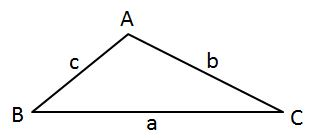#### Question 22 22. What is the following radian measure in degrees?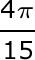#### Question 23 23. Which of these angles can replace x in tan (x)?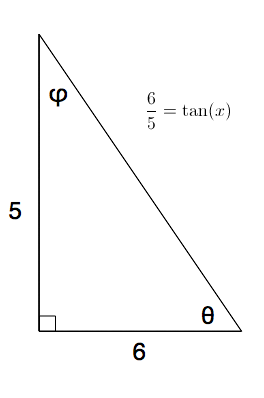#### Question 24 24. Solve the following equation for x.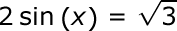#### Question 25 25. What is z in terms of x and y?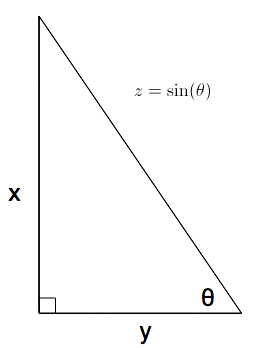### Page 6

#### Question 26 26. Which tangent function would result in the graph below?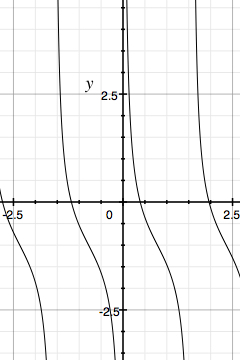#### Question 28 28. What is the value of cos(θ) given that the equation below is true?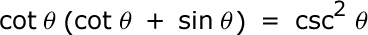#### Question 30 30. What is z in terms of x?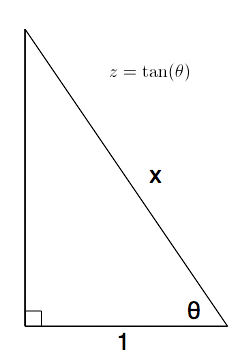#### Overview of Trigonometry: Help and Review Chapter Exam Instructions

Choose your answers to the questions and click 'Next' to see the next set of questions. You can skip questions if you would like and come back to them later with the yellow "Go To First Skipped Question" button. When you have completed the practice exam, a green submit button will appear. Click it to see your results. Good luck!

Support